Data Whitening¶

A whitening transformation is a decorrelation transformation that transforms a set of random variables into a set of new random variables with identity covariance (uncorrelated with unit variances).

In particular, suppose a random vector has covariance, then a whitening transform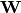is one that satisfy: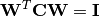Note thatis generally not unique. In particular, ifis a whitening transform, so is any of its rotation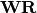with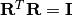.

Whitening¶

The package uses Whitening defined below to represent a whitening transform:

immutable Whitening{T<:FloatingPoint}
mean::Vector{T}     # mean vector (can be empty to indicate zero mean), of length d
W::Matrix{T}        # the transform coefficient matrix, of size (d, d)
end

An instance of Whitening can be constructed by Whitening(mean, W).

There are several functions to access the properties of a whitening transform f:

indim(f)

Get the input dimension, i.e d.

outdim(f)

Get the out dimension, i.e d.

mean(f)

Get the mean vector.

Note: if f.mean is empty, this function returns a zero vector of length d.

transform(f, x)

Apply the whitening transform to a vector or a matrix with samples in columns, as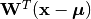.

Data Analysis¶

Given a dataset, one can use the fit method to estimate a whitening transform.

fit(Whitening, X; ...)

Estimate a whitening transform from the data given in X. Here, X should be a matrix, whose columns give the samples.

This function returns an instance of Whitening.

Keyword Arguments:

name description default
regcoef

The regularization coefficient. The covariance will be regularized as follows when regcoef is positive:

C + (eigmax(C) * regcoef) * eye(d)

zero(T)
mean

The mean vector, which can be either of:

• 0: the input data has already been centralized
• nothing: this function will compute the mean
• a pre-computed mean vector
nothing

Note: This function internally relies on cov_whiten to derive the transformation W. The function cov_whiten itself is also a useful function.

cov_whitening(C)

Derive the whitening transform coefficient matrix W given the covariance matrix C. Here, C can be either a square matrix, or an instance of Cholesky.

Internally, this function solves the whitening transform using Cholesky factorization. The rationale is as follows: let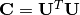and, then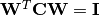.

Note: The return matrix W is an upper triangular matrix.

cov_whitening(C, regcoef)

Derive a whitening transform based on a regularized covariance, as C + (eigmax(C) * regcoef) * eye(d).

In addition, the package also provides cov_whiten!, in which the input matrix C will be overwritten during computation. This can be more efficient when C is no longer used.

invsqrtm(C)

Compute inv(sqrtm(C)) through symmetric eigenvalue decomposition.